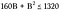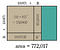# The Lost Art of Square Roots

You’ve probably never done this before. In fact for a long time it never even crossed my mind that I hadn’t a clue how to.

It was all purecalculator magic ✨.

The calculator knows the answer. I don’t know how, but it does. And well, that’s where the story ended.

Good enough, yeah?

But this is about so much more than solving an already solved problem.

It’s about finding beauty in the mundane. About understanding something not because you have to, but because you can. And it’s about seeing how every ounce of mathematics has been fought for, discovered, and sifted from a greater logical system.

Ready to take a quick trip through the lost art of solving square roots?

# How to Solve Square Roots by Hand

I could show you a great estimation technique, but I’m not going to.

Although estimation is a wonderful, practical tool, I’m here to show you a lost art. And even better than that I’m going to take this antiquated algorithm, blow the dust off, and give it to you in pictures.

Because seeing is believing, and more importantly seeing is remembering.

So what are we waiting for? Let’s go!

# Getting Started

Our algorithm requires us to work with two digits at a time, therefore we must begin with an even quantity of digits.

If you have an odd number of digits, we need to adjust it first.

• If there are an odd number of digits before the decimal place, add a zero to the front of the number, like this:
• If there are an odd number of digits after the decimal place, add a zero to the end, like this:

# Solving The Problem

Alright, let’s find the square root of 7720.17.

# Step 0: Check the Digits

7720.17 has six digits total, so we’re good to go!

# Step 1: Make an Area Diagram

Draw a rectangle to represent the area 7720.17.

Add a square inside the rectangle.

# Step 2: Split the Tens and Ones

We know a square has sides of equal length. Instead of writing a variable to represent these lengths, we’ll divide the unknown lengths into a tens part and ones part.

Let 10A represent the tens and B represent the ones, so that the unknown side length is (10A + B).

Go ahead and subdivide the square and fill in the areas of the new sections by multiplying together the respective side lengths.

The area of the entire tan square is the sum of its parts:

# Step 3 : Solve For “A”

We know the area of the 10A square is less than/equal to the whole rectangle.

Divide by 10² and ignore everything after the first two digits.

The answer for A will be the largest whole number that fits this equation:

## Note

Dividing by 10² happens to work out nicely in this case. In general, you can simply set the first 2 digits of the number greater than/equal to A².

8² is the closest we can get to 77. So 8 will be the first digit of our answer. While you’re at it subtract 8² from 77 and bring down the next 2 digits.

Let’s fill in our diagram with A=8.

We’ve accounted for 6400 of 7720.17 square units. The remaining area must be less than the difference of 1320.17 square units.

# Step 4 : Find the Remaining Area

We have three remaining sections which involve B. We know they cannot exceed 1320.17 square units.

Add together the remaining sections and set them less than/equal to 1320, the value we found in step 3.Notice we have two 10(8)B areas, so that totals to 160B.

Factor out B.

Now all we have to do is think of a single digit number that gets us close to 1320.

With a little trial, we find that when B=7 we get close to 1320.

Subtract 1169 from 1320 and bring down the next two digits.

# Step 5: Magnify and Repeat

We’ve reached the decimal point, which means we’ve finished finding the whole number part of our answer! Now we’ll work on adding precision.

By not bringing down the decimal point with 17, we’re essentially magnifying the area by 100. This calls for a new diagram, this time with an area of 772,017 instead of 7720.17.

In this new model, A is the square root answer thus far, so A=87.Note: You needn’t multiply (10•87)² to solve the problem. I just did for demonstration.

The next digit in our solution will be the answer to B in this new diagram. The remaining areas involving B are less than/equal to 15,117 (from step 4).

After summing the areas and factoring out B, we get:

Again we’re looking for a single digit number that gets us closest to 15,117. Using estimation, we find that plugging in B=8 gets us close at 13,984.

So the next digit in our answer is 8.

It’s easy to calculate as many digits as you like.

After you subtract the previous area, bring down the next two digits. When you run out of digits simply add two zeros to the end and bring them down instead.

Once you do this, set up another diagram (which turns out to be a magnitude of 100 larger than the previous). The “new B” you solve for will be the next digit in the solution.

Rinse & repeat!

Boom!

That’s it.

Square roots by hand — a totally unnecessary, but awesome thing to know!

Personally, I love using diagrams. I know that if sometime down the road someone asks me to calculate a square root, I don’t have to depend purely on my memory to remember the steps.

Instead I can recreate the steps using the basic premises, a little understanding, and some logic.

## ❤ STAY CONNECTED ❤

Stay up-to-date with everything Math Hacks is up to!

## Math Hacks

Tutorials with a fresh perspective.

Written by

## Brett Berry

Check out my YouTube channel “Math Hacks” for hands-on math tutorials and lots of math love ♥️## Math Hacks

Tutorials with a fresh perspective.